# RD Sharma Solutions for Class 6 Maths Chapter 6: Fractions Exercise 6.9

Unlike fractions are fractions with different denominators. This exercise has problems on addition and subtraction of unlike fractions. RD Sharma Solutions Class 6 are well structured and designed relevant to the marks weightage in the Class 6 exam. The teachers at BYJU’S prepare solutions in order to make the concepts easy for the students to understand. The students can get an idea about the other methods which can be followed to solve the complex problems in a shorter duration. The exercise wise problems present in the textbook can be understood more easily with the help of RD Sharma Solutions for Class 6 Maths Chapter 6 Fractions Exercise 6.9 PDF which are provided here.

## RD Sharma Solutions for Class 6 Maths Chapter 6: Fractions Exercise 6.9 Download PDF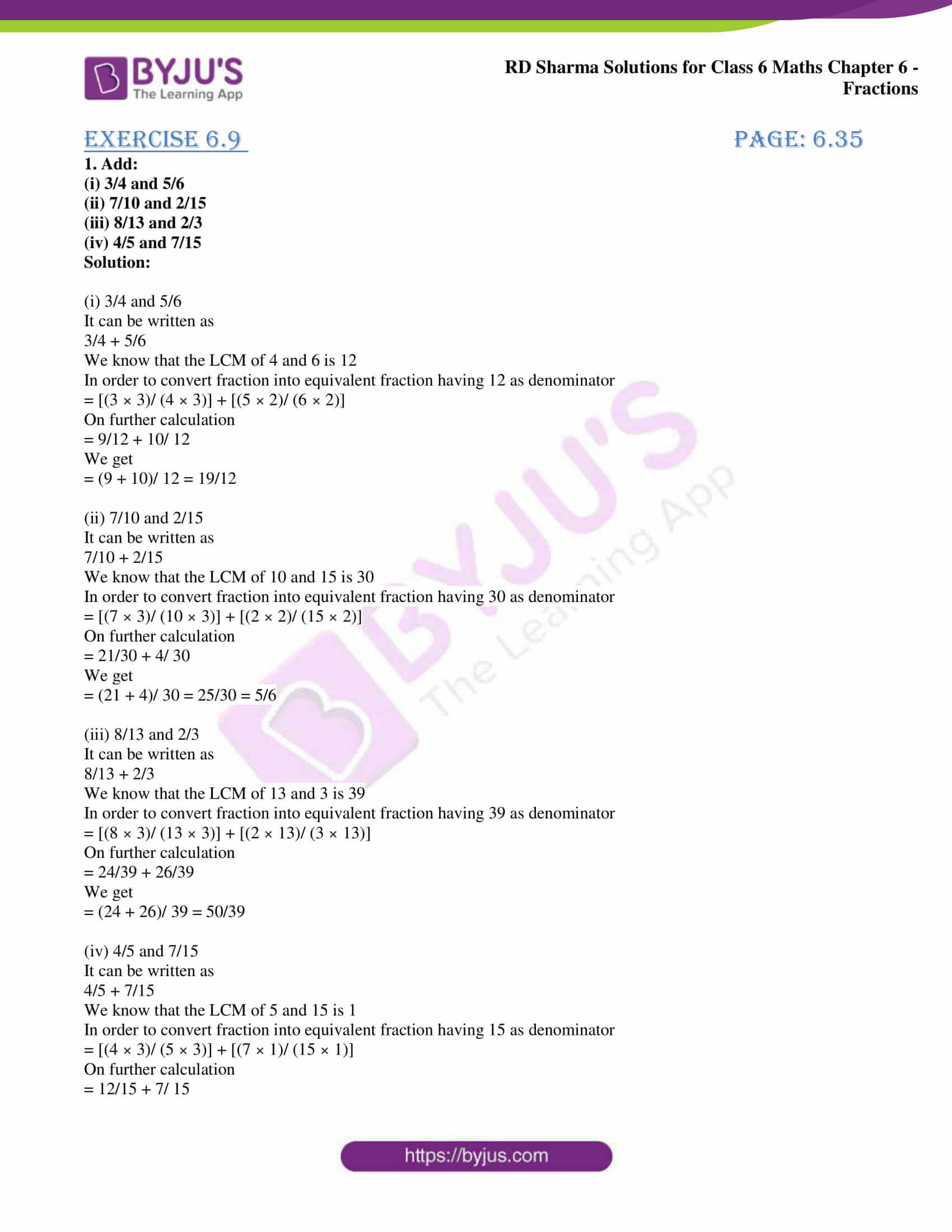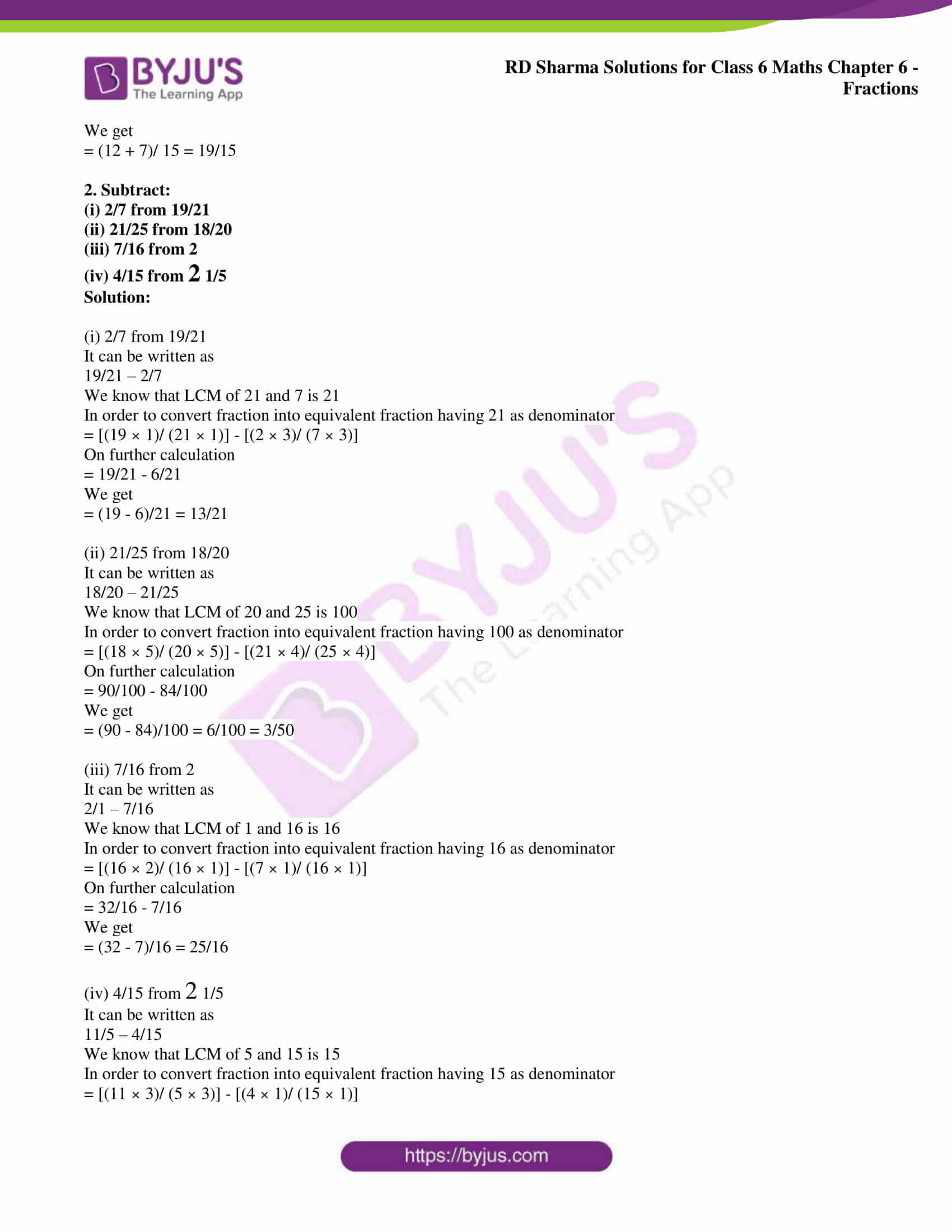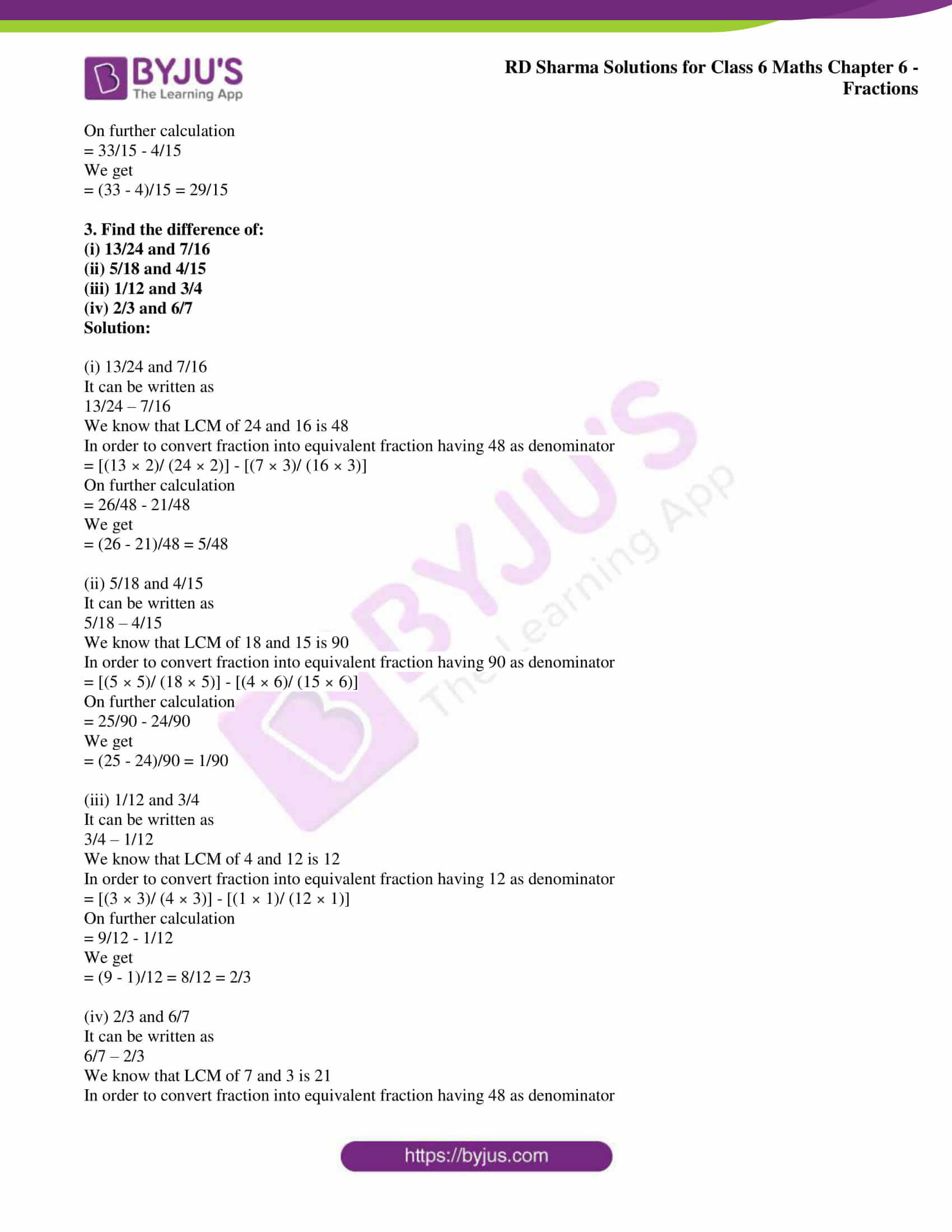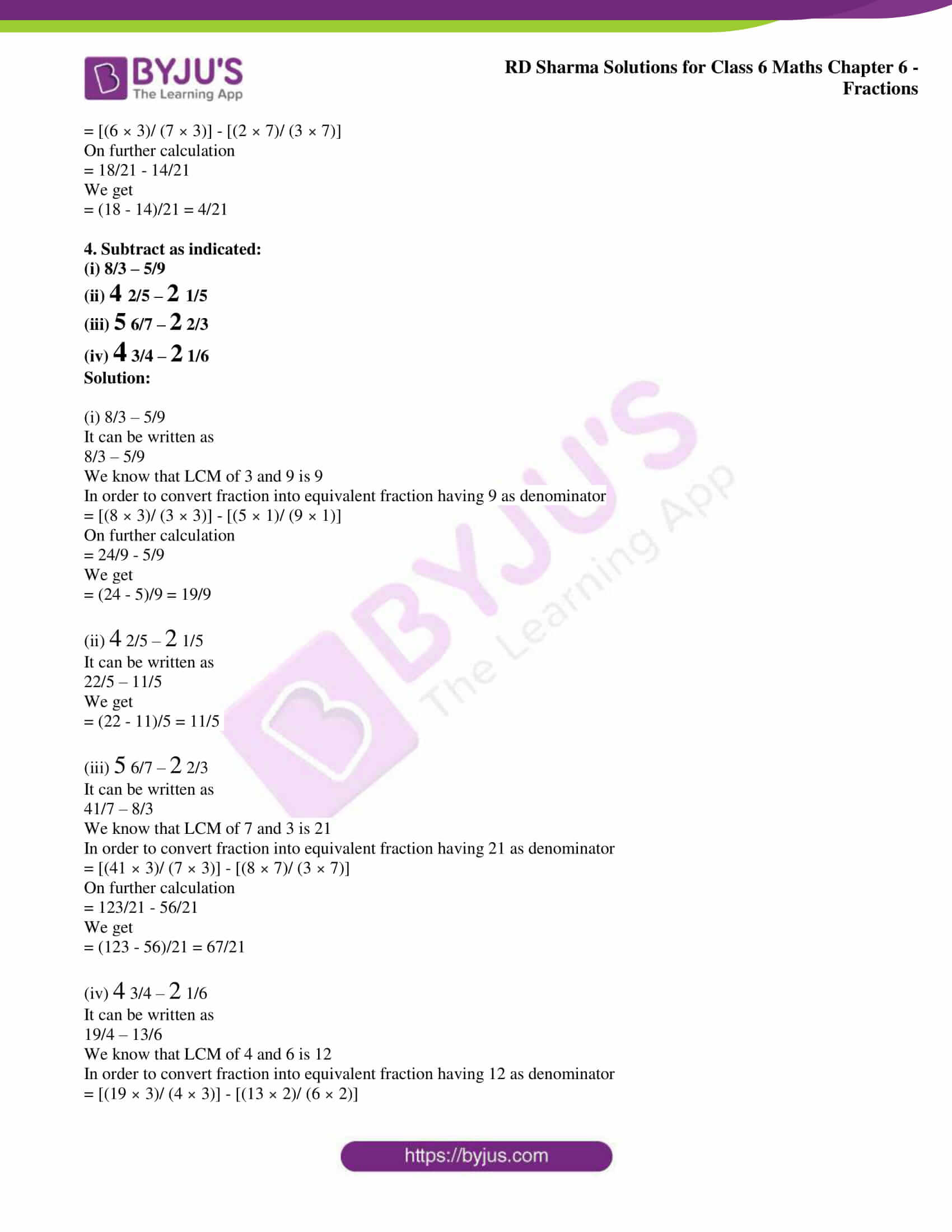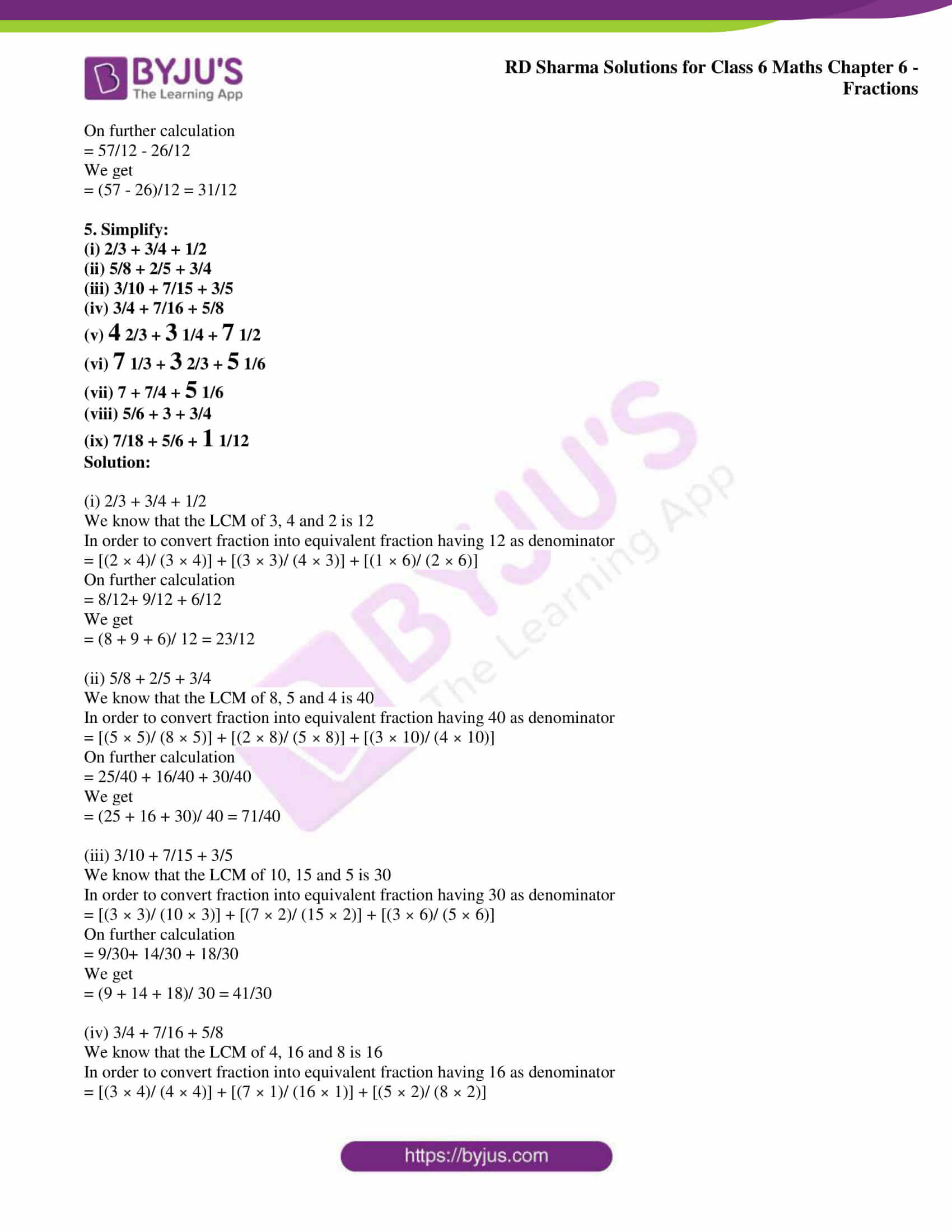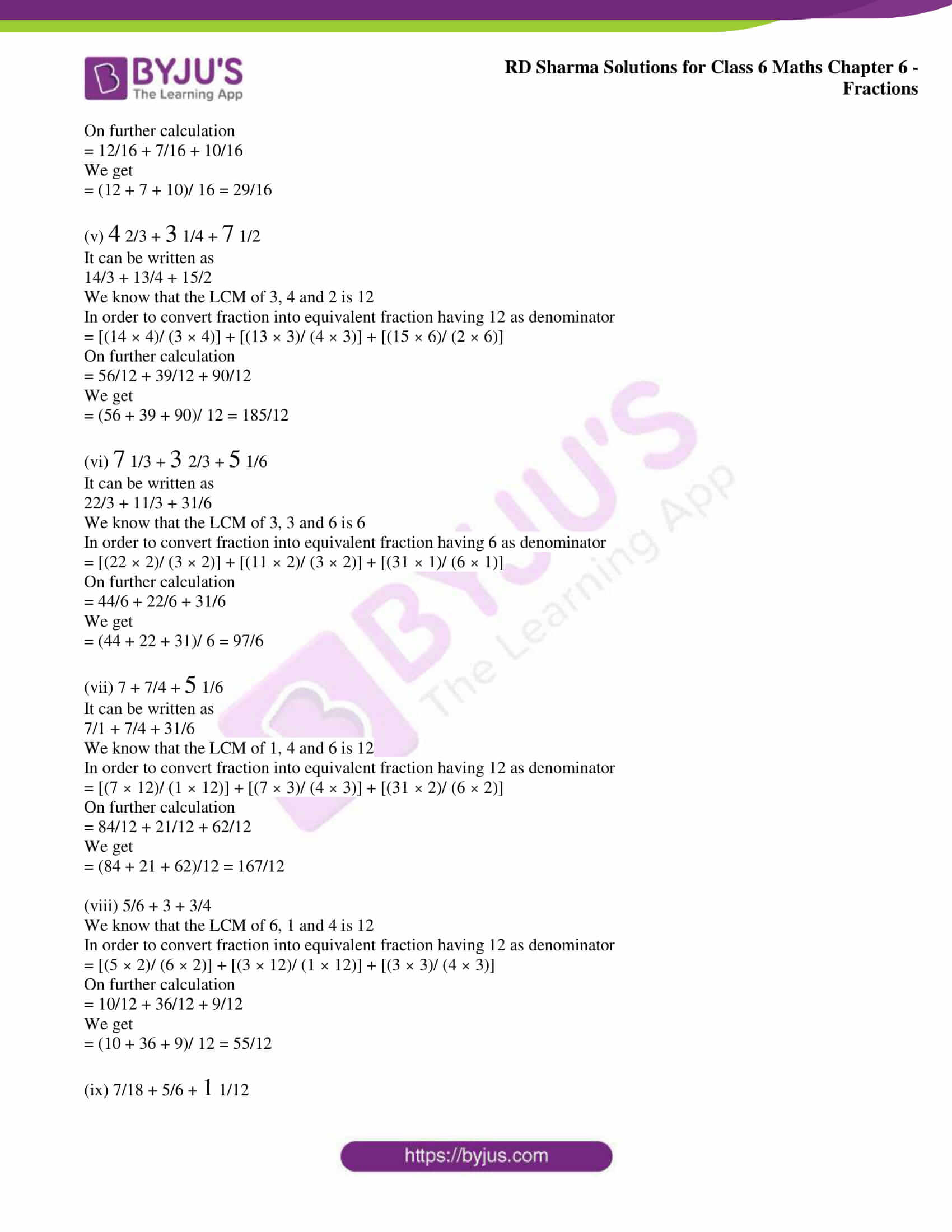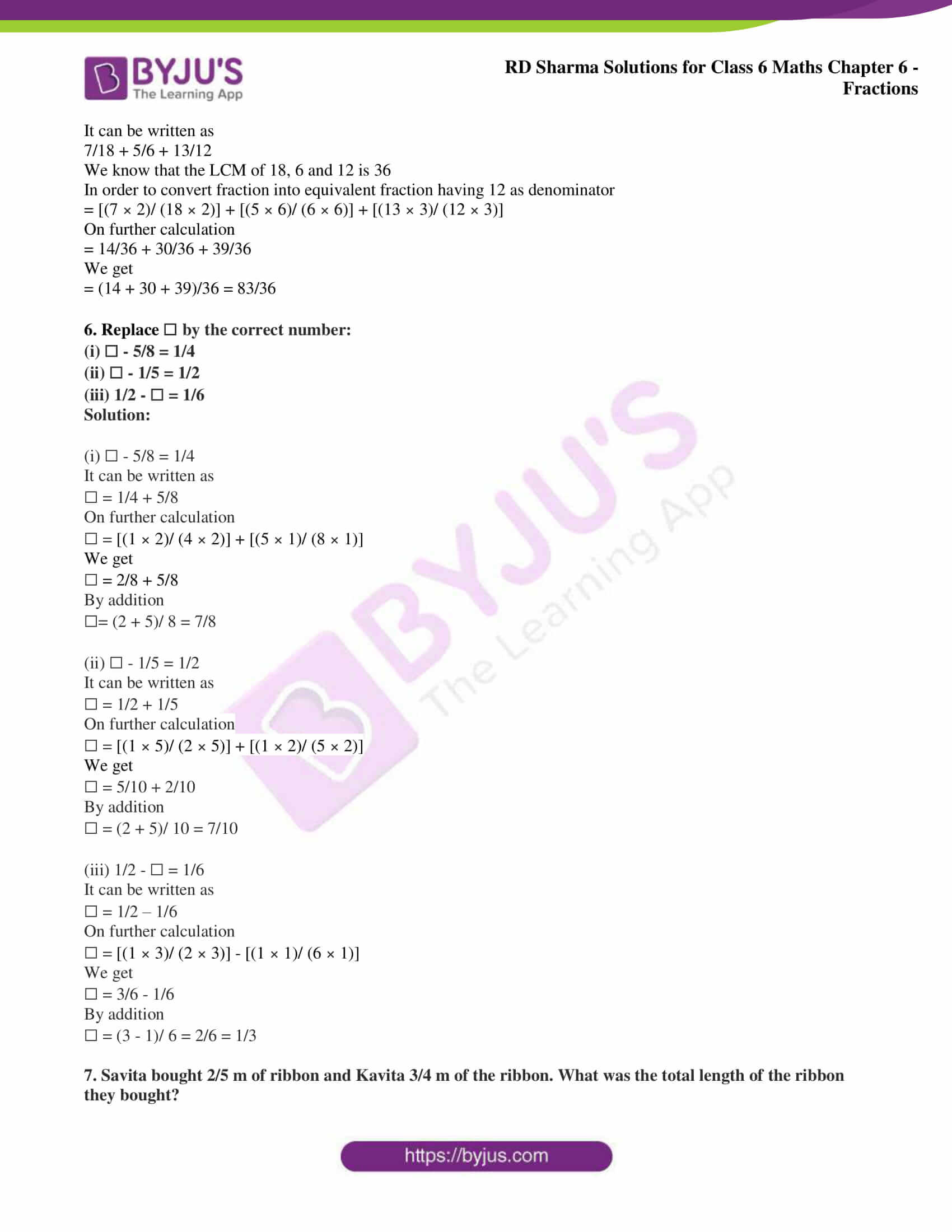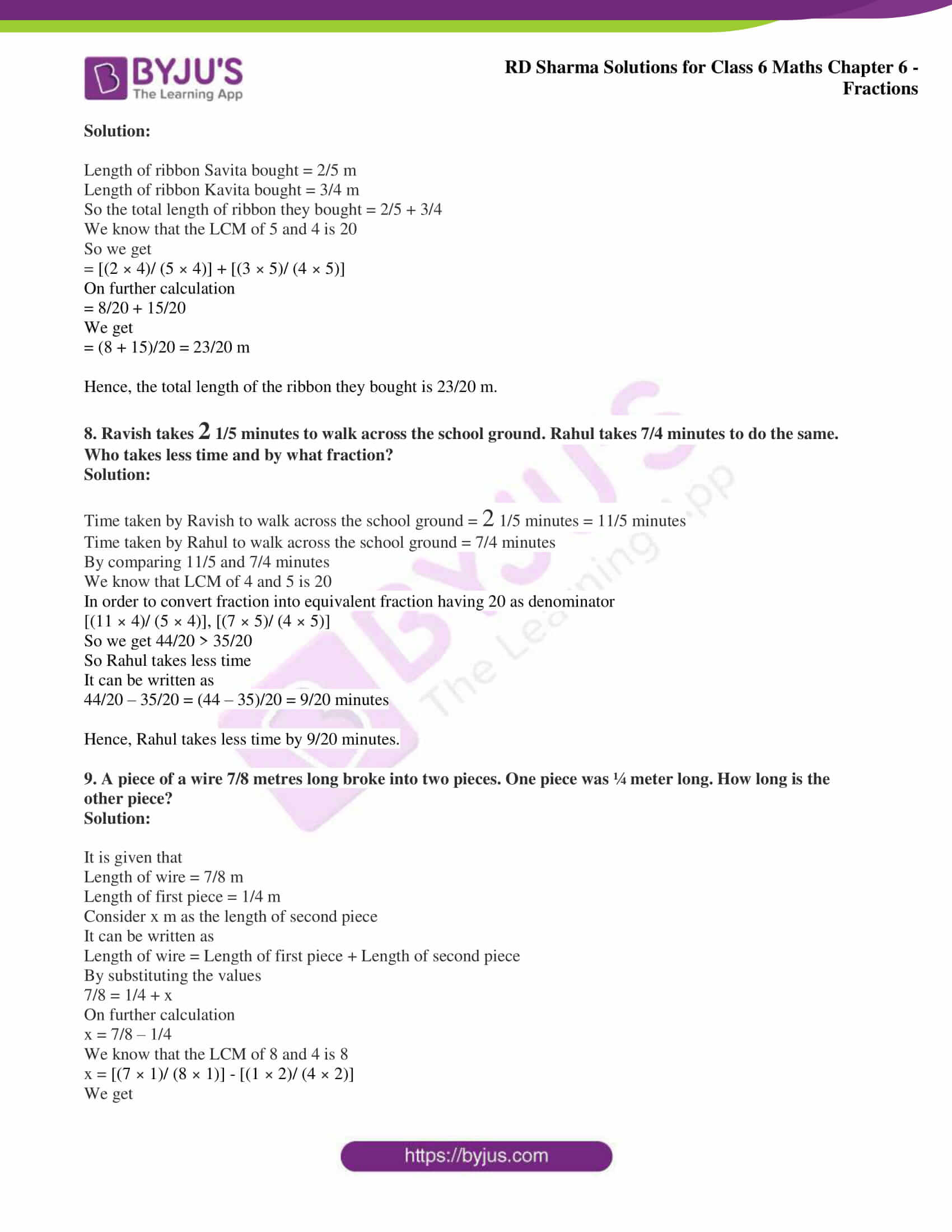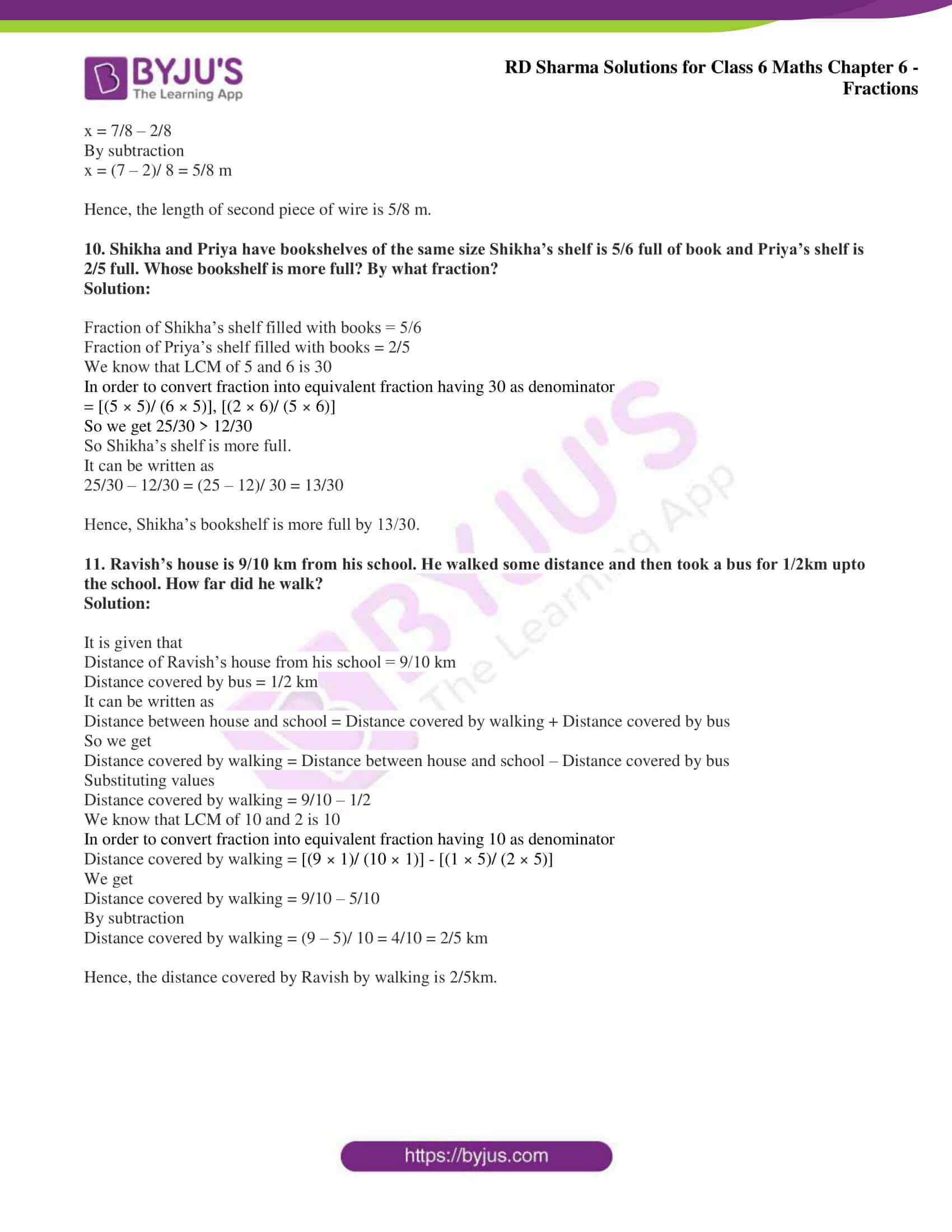### Access answers to Maths RD Sharma Solutions for Class 6 Chapter 6: Fractions Exercise 6.9

(i) 3/4 and 5/6

(ii) 7/10 and 2/15

(iii) 8/13 and 2/3

(iv) 4/5 and 7/15

Solution:

(i) 3/4 and 5/6

It can be written as

3/4 + 5/6

We know that the LCM of 4 and 6 is 12

In order to convert fraction into equivalent fraction having 12 as denominator

= [(3 × 3)/ (4 × 3)] + [(5 × 2)/ (6 × 2)]

On further calculation

= 9/12 + 10/ 12

We get

= (9 + 10)/ 12 = 19/12

(ii) 7/10 and 2/15

It can be written as

7/10 + 2/15

We know that the LCM of 10 and 15 is 30

In order to convert fraction into equivalent fraction having 30 as denominator

= [(7 × 3)/ (10 × 3)] + [(2 × 2)/ (15 × 2)]

On further calculation

= 21/30 + 4/ 30

We get

= (21 + 4)/ 30 = 25/30 = 5/6

(iii) 8/13 and 2/3

It can be written as

8/13 + 2/3

We know that the LCM of 13 and 3 is 39

In order to convert fraction into equivalent fraction having 39 as denominator

= [(8 × 3)/ (13 × 3)] + [(2 × 13)/ (3 × 13)]

On further calculation

= 24/39 + 26/39

We get

= (24 + 26)/ 39 = 50/39

(iv) 4/5 and 7/15

It can be written as

4/5 + 7/15

We know that the LCM of 5 and 15 is 1

In order to convert fraction into equivalent fraction having 15 as denominator

= [(4 × 3)/ (5 × 3)] + [(7 × 1)/ (15 × 1)]

On further calculation

= 12/15 + 7/ 15

We get

= (12 + 7)/ 15 = 19/15

2. Subtract:

(i) 2/7 from 19/21

(ii) 21/25 from 18/20

(iii) 7/16 from 2

(iv) 4/15 from 2 1/5

Solution:

(i) 2/7 from 19/21

It can be written as

19/21 – 2/7

We know that LCM of 21 and 7 is 21

In order to convert fraction into equivalent fraction having 21 as denominator

= [(19 × 1)/ (21 × 1)] – [(2 × 3)/ (7 × 3)]

On further calculation

= 19/21 – 6/21

We get

= (19 – 6)/21 = 13/21

(ii) 21/25 from 18/20

It can be written as

18/20 – 21/25

We know that LCM of 20 and 25 is 100

In order to convert fraction into equivalent fraction having 100 as denominator

= [(18 × 5)/ (20 × 5)] – [(21 × 4)/ (25 × 4)]

On further calculation

= 90/100 – 84/100

We get

= (90 – 84)/100 = 6/100 = 3/50

(iii) 7/16 from 2

It can be written as

2/1 – 7/16

We know that LCM of 1 and 16 is 16

In order to convert fraction into equivalent fraction having 16 as denominator

= [(16 × 2)/ (16 × 1)] – [(7 × 1)/ (16 × 1)]

On further calculation

= 32/16 – 7/16

We get

= (32 – 7)/16 = 25/16

(iv) 4/15 from 2 1/5

It can be written as

11/5 – 4/15

We know that LCM of 5 and 15 is 15

In order to convert fraction into equivalent fraction having 15 as denominator

= [(11 × 3)/ (5 × 3)] – [(4 × 1)/ (15 × 1)]

On further calculation

= 33/15 – 4/15

We get

= (33 – 4)/15 = 29/15

3. Find the difference of:

(i) 13/24 and 7/16

(ii) 5/18 and 4/15

(iii) 1/12 and 3/4

(iv) 2/3 and 6/7

Solution:

(i) 13/24 and 7/16

It can be written as

13/24 – 7/16

We know that LCM of 24 and 16 is 48

In order to convert fraction into equivalent fraction having 48 as denominator

= [(13 × 2)/ (24 × 2)] – [(7 × 3)/ (16 × 3)]

On further calculation

= 26/48 – 21/48

We get

= (26 – 21)/48 = 5/48

(ii) 5/18 and 4/15

It can be written as

5/18 – 4/15

We know that LCM of 18 and 15 is 90

In order to convert fraction into equivalent fraction having 90 as denominator

= [(5 × 5)/ (18 × 5)] – [(4 × 6)/ (15 × 6)]

On further calculation

= 25/90 – 24/90

We get

= (25 – 24)/90 = 1/90

(iii) 1/12 and 3/4

It can be written as

3/4 – 1/12

We know that LCM of 4 and 12 is 12

In order to convert fraction into equivalent fraction having 12 as denominator

= [(3 × 3)/ (4 × 3)] – [(1 × 1)/ (12 × 1)]

On further calculation

= 9/12 – 1/12

We get

= (9 – 1)/12 = 8/12 = 2/3

(iv) 2/3 and 6/7

It can be written as

6/7 – 2/3

We know that LCM of 7 and 3 is 21

In order to convert fraction into equivalent fraction having 48 as denominator

= [(6 × 3)/ (7 × 3)] – [(2 × 7)/ (3 × 7)]

On further calculation

= 18/21 – 14/21

We get

= (18 – 14)/21 = 4/21

4. Subtract as indicated:

(i) 8/3 – 5/9

(ii) 4 2/5 – 2 1/5

(iii) 5 6/7 – 2 2/3

(iv) 4 3/4 – 2 1/6

Solution:

(i) 8/3 – 5/9

It can be written as

8/3 – 5/9

We know that LCM of 3 and 9 is 9

In order to convert fraction into equivalent fraction having 9 as denominator

= [(8 × 3)/ (3 × 3)] – [(5 × 1)/ (9 × 1)]

On further calculation

= 24/9 – 5/9

We get

= (24 – 5)/9 = 19/9

(ii) 4 2/5 – 2 1/5

It can be written as

22/5 – 11/5

We get

= (22 – 11)/5 = 11/5

(iii) 5 6/7 – 2 2/3

It can be written as

41/7 – 8/3

We know that LCM of 7 and 3 is 21

In order to convert fraction into equivalent fraction having 21 as denominator

= [(41 × 3)/ (7 × 3)] – [(8 × 7)/ (3 × 7)]

On further calculation

= 123/21 – 56/21

We get

= (123 – 56)/21 = 67/21

(iv) 4 3/4 – 2 1/6

It can be written as

19/4 – 13/6

We know that LCM of 4 and 6 is 12

In order to convert fraction into equivalent fraction having 12 as denominator

= [(19 × 3)/ (4 × 3)] – [(13 × 2)/ (6 × 2)]

On further calculation

= 57/12 – 26/12

We get

= (57 – 26)/12 = 31/12

5. Simplify:

(i) 2/3 + 3/4 + 1/2

(ii) 5/8 + 2/5 + 3/4

(iii) 3/10 + 7/15 + 3/5

(iv) 3/4 + 7/16 + 5/8

(v) 4 2/3 + 3 1/4 + 7 1/2

(vi) 7 1/3 + 3 2/3 + 5 1/6

(vii) 7 + 7/4 + 5 1/6

(viii) 5/6 + 3 + 3/4

(ix) 7/18 + 5/6 + 1 1/12

Solution:

(i) 2/3 + 3/4 + 1/2

We know that the LCM of 3, 4 and 2 is 12

In order to convert fraction into equivalent fraction having 12 as denominator

= [(2 × 4)/ (3 × 4)] + [(3 × 3)/ (4 × 3)] + [(1 × 6)/ (2 × 6)]

On further calculation

= 8/12+ 9/12 + 6/12

We get

= (8 + 9 + 6)/ 12 = 23/12

(ii) 5/8 + 2/5 + 3/4

We know that the LCM of 8, 5 and 4 is 40

In order to convert fraction into equivalent fraction having 40 as denominator

= [(5 × 5)/ (8 × 5)] + [(2 × 8)/ (5 × 8)] + [(3 × 10)/ (4 × 10)]

On further calculation

= 25/40 + 16/40 + 30/40

We get

= (25 + 16 + 30)/ 40 = 71/40

(iii) 3/10 + 7/15 + 3/5

We know that the LCM of 10, 15 and 5 is 30

In order to convert fraction into equivalent fraction having 30 as denominator

= [(3 × 3)/ (10 × 3)] + [(7 × 2)/ (15 × 2)] + [(3 × 6)/ (5 × 6)]

On further calculation

= 9/30+ 14/30 + 18/30

We get

= (9 + 14 + 18)/ 30 = 41/30

(iv) 3/4 + 7/16 + 5/8

We know that the LCM of 4, 16 and 8 is 16

In order to convert fraction into equivalent fraction having 16 as denominator

= [(3 × 4)/ (4 × 4)] + [(7 × 1)/ (16 × 1)] + [(5 × 2)/ (8 × 2)]

On further calculation

= 12/16 + 7/16 + 10/16

We get

= (12 + 7 + 10)/ 16 = 29/16

(v) 4 2/3 + 3 1/4 + 7 1/2

It can be written as

14/3 + 13/4 + 15/2

We know that the LCM of 3, 4 and 2 is 12

In order to convert fraction into equivalent fraction having 12 as denominator

= [(14 × 4)/ (3 × 4)] + [(13 × 3)/ (4 × 3)] + [(15 × 6)/ (2 × 6)]

On further calculation

= 56/12 + 39/12 + 90/12

We get

= (56 + 39 + 90)/ 12 = 185/12

(vi) 7 1/3 + 3 2/3 + 5 1/6

It can be written as

22/3 + 11/3 + 31/6

We know that the LCM of 3, 3 and 6 is 6

In order to convert fraction into equivalent fraction having 6 as denominator

= [(22 × 2)/ (3 × 2)] + [(11 × 2)/ (3 × 2)] + [(31 × 1)/ (6 × 1)]

On further calculation

= 44/6 + 22/6 + 31/6

We get

= (44 + 22 + 31)/ 6 = 97/6

(vii) 7 + 7/4 + 5 1/6

It can be written as

7/1 + 7/4 + 31/6

We know that the LCM of 1, 4 and 6 is 12

In order to convert fraction into equivalent fraction having 12 as denominator

= [(7 × 12)/ (1 × 12)] + [(7 × 3)/ (4 × 3)] + [(31 × 2)/ (6 × 2)]

On further calculation

= 84/12 + 21/12 + 62/12

We get

= (84 + 21 + 62)/12 = 167/12

(viii) 5/6 + 3 + 3/4

We know that the LCM of 6, 1 and 4 is 12

In order to convert fraction into equivalent fraction having 12 as denominator

= [(5 × 2)/ (6 × 2)] + [(3 × 12)/ (1 × 12)] + [(3 × 3)/ (4 × 3)]

On further calculation

= 10/12 + 36/12 + 9/12

We get

= (10 + 36 + 9)/ 12 = 55/12

(ix) 7/18 + 5/6 + 1 1/12

It can be written as

7/18 + 5/6 + 13/12

We know that the LCM of 18, 6 and 12 is 36

In order to convert fraction into equivalent fraction having 12 as denominator

= [(7 × 2)/ (18 × 2)] + [(5 × 6)/ (6 × 6)] + [(13 × 3)/ (12 × 3)]

On further calculation

= 14/36 + 30/36 + 39/36

We get

= (14 + 30 + 39)/36 = 83/36

6. Replace ☐ by the correct number:

(i) ☐ – 5/8 = 1/4

(ii) ☐ – 1/5 = 1/2

(iii) 1/2 – ☐ = 1/6

Solution:

(i) ☐ – 5/8 = 1/4

It can be written as

☐ = 1/4 + 5/8

On further calculation

☐ = [(1 × 2)/ (4 × 2)] + [(5 × 1)/ (8 × 1)]

We get

☐ = 2/8 + 5/8

☐= (2 + 5)/ 8 = 7/8

(ii) ☐ – 1/5 = 1/2

It can be written as

☐ = 1/2 + 1/5

On further calculation

☐ = [(1 × 5)/ (2 × 5)] + [(1 × 2)/ (5 × 2)]

We get

☐ = 5/10 + 2/10

☐ = (2 + 5)/ 10 = 7/10

(iii) 1/2 – ☐ = 1/6

It can be written as

☐ = 1/2 – 1/6

On further calculation

☐ = [(1 × 3)/ (2 × 3)] – [(1 × 1)/ (6 × 1)]

We get

☐ = 3/6 – 1/6

☐ = (3 – 1)/ 6 = 2/6 = 1/3

7. Savita bought 2/5 m of ribbon and Kavita 3/4 m of the ribbon. What was the total length of the ribbon they bought?

Solution:

Length of ribbon Savita bought = 2/5 m

Length of ribbon Kavita bought = 3/4 m

So the total length of ribbon they bought = 2/5 + 3/4

We know that the LCM of 5 and 4 is 20

So we get

= [(2 × 4)/ (5 × 4)] + [(3 × 5)/ (4 × 5)]

On further calculation

= 8/20 + 15/20

We get

= (8 + 15)/20 = 23/20 m

Hence, the total length of the ribbon they bought is 23/20 m.

8. Ravish takes 2 1/5 minutes to walk across the school ground. Rahul takes 7/4 minutes to do the same. Who takes less time and by what fraction?

Solution:

Time taken by Ravish to walk across the school ground = 2 1/5 minutes = 11/5 minutes

Time taken by Rahul to walk across the school ground = 7/4 minutes

By comparing 11/5 and 7/4 minutes

We know that LCM of 4 and 5 is 20

In order to convert fraction into equivalent fraction having 20 as denominator

[(11 × 4)/ (5 × 4)], [(7 × 5)/ (4 × 5)]

So we get 44/20 > 35/20

So Rahul takes less time

It can be written as

44/20 – 35/20 = (44 – 35)/20 = 9/20 minutes

Hence, Rahul takes less time by 9/20 minutes.

9. A piece of a wire 7/8 metres long broke into two pieces. One piece was ¼ meter long. How long is the other piece?

Solution:

It is given that

Length of wire = 7/8 m

Length of first piece = 1/4 m

Consider x m as the length of second piece

It can be written as

Length of wire = Length of first piece + Length of second piece

By substituting the values

7/8 = 1/4 + x

On further calculation

x = 7/8 – 1/4

We know that the LCM of 8 and 4 is 8

x = [(7 × 1)/ (8 × 1)] – [(1 × 2)/ (4 × 2)]

We get

x = 7/8 – 2/8

By subtraction

x = (7 – 2)/ 8 = 5/8 m

Hence, the length of second piece of wire is 5/8 m.

10. Shikha and Priya have bookshelves of the same size Shikha’s shelf is 5/6 full of book and Priya’s shelf is 2/5 full. Whose bookshelf is more full? By what fraction?

Solution:

Fraction of Shikha’s shelf filled with books = 5/6

Fraction of Priya’s shelf filled with books = 2/5

We know that LCM of 5 and 6 is 30

In order to convert fraction into equivalent fraction having 30 as denominator

= [(5 × 5)/ (6 × 5)], [(2 × 6)/ (5 × 6)]

So we get 25/30 > 12/30

So Shikha’s shelf is more full.

It can be written as

25/30 – 12/30 = (25 – 12)/ 30 = 13/30

Hence, Shikha’s bookshelf is more full by 13/30.

11. Ravish’s house is 9/10 km from his school. He walked some distance and then took a bus for 1/2km upto the school. How far did he walk?

Solution:

It is given that

Distance of Ravish’s house from his school = 9/10 km

Distance covered by bus = 1/2 km

It can be written as

Distance between house and school = Distance covered by walking + Distance covered by bus

So we get

Distance covered by walking = Distance between house and school – Distance covered by bus

Substituting values

Distance covered by walking = 9/10 – 1/2

We know that LCM of 10 and 2 is 10

In order to convert fraction into equivalent fraction having 10 as denominator

Distance covered by walking = [(9 × 1)/ (10 × 1)] – [(1 × 5)/ (2 × 5)]

We get

Distance covered by walking = 9/10 – 5/10

By subtraction

Distance covered by walking = (9 – 5)/ 10 = 4/10 = 2/5 km

Hence, the distance covered by Ravish by walking is 2/5km.